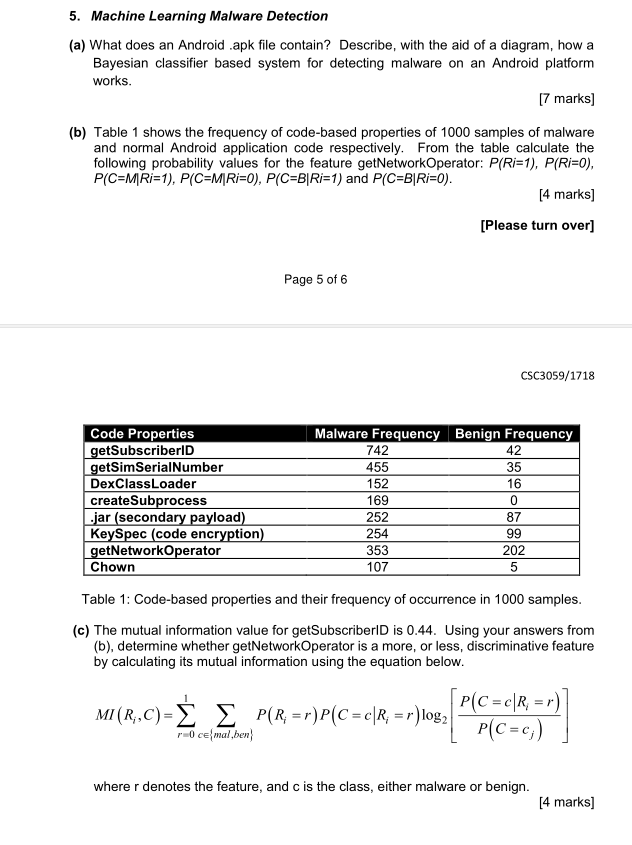# 5 machine learning malware detection a what does an android apk file contain describ 51494145. Machine Learning Malware Detection (a) What does an Android .apk file contain? Describe, with the aid of a diagram, how a Bayesian classifier based system for detecting malware on an Android platform works [7 marks] (b) Table 1 shows the frequency of code-based properties of 1000 samples of malware and normal Android application code respectively. From the table calculate the following probability values for the feature getNetworkOperator: P(Ri=1), P(Ri=0), P(C=MRi=1), P(C=M|Ri=0), P(C=B|Ri=1) and P(C-B|Ri=0) [4 marks] [Please turn over] Page 5 of 6 CSC3059/1718 Malware Frequency Benign Frequency Code Properties getSubscriberID getSimSerialNumber DexClassLoader createSubprocess jar (secondary payload) KeySpec (code encryption) getNetworkOperator Chown 742 42 455 35 16 152 169 C 252 254 87 99 353 202 107 5 Table 1: Code-based properties and their frequency of occurrence in 1000 samples. (c) The mutual information value for getSubscriberlD is 0.44. Using your answers from (b), determine whether getNetworkOperator is a more, or less, discriminative feature by calculating its mutual information using the equation below. P(C=cR, =r) P(C=c) P(Rr)P(C cR r) log2 M (R, C) – Σ Σ 0cemal,ben where r denotes the feature, and c is the class, either malware or benign [4 marks]